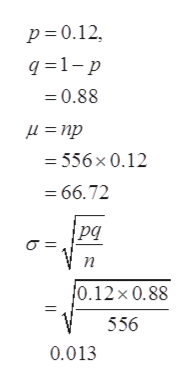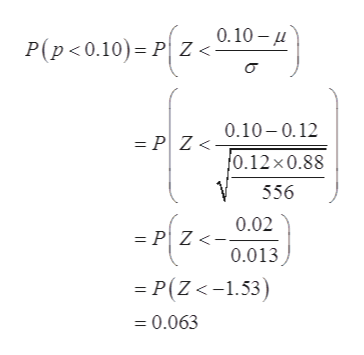# A hotel manager calculates that 12% of the hotel rooms are booked.If the manager is right, what is the probability that the proportion of rooms booked in a sample of 556 rooms would be less than 10%? Round your answer to four decimal places.

Question
36 views

A hotel manager calculates that 12% of the hotel rooms are booked.

If the manager is right, what is the probability that the proportion of rooms booked in a sample of 556 rooms would be less than 10%? Round your answer to four decimal places.

check_circle

Step 1

From the given information,

P be the probability that hotel rooms are booked = 0.12.

Sample size (n) =556,

By normal approximation of binomial,

Thus,help_outlineImage Transcriptionclosep 0.12 q 1p =0.88 =556x 0.12 =66.72 рg п 0.12x 0.88 556 0.013 fullscreen
Step 2

So, probability that the proportion of rooms booked in a sample...help_outlineImage Transcriptionclose0.10- P(p<0.10) PZ<- 0.10-0.12 = PZ < 0.12 x0.88 556 0.02 = P Z<- 0.013 P(Z <-1.53) = 0.063 fullscreen

### Want to see the full answer?

See Solution

#### Want to see this answer and more?

Solutions are written by subject experts who are available 24/7. Questions are typically answered within 1 hour.*

See Solution
*Response times may vary by subject and question.
Tagged in

### Statistics